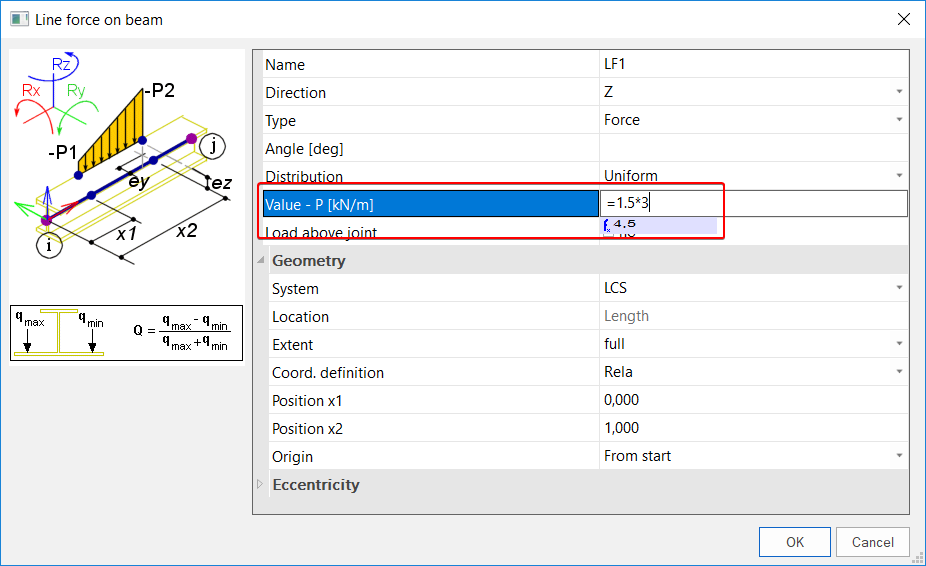Menu

# Is it possible to perform calculation in the value fields of loads?

While defining loads on a structure, it might be necessary to perform a small calculation in order to determine the exact value of the load. For example, when a wind load of 1,5 kN/m² acts on the side of a pilot with a frame distance of 3 m, the resulting line load of a column will be 4,5 kN/m. Instead of using a calculator, SCIA can enter the equating itself.

In order to perform this small calculation, the equation in the value field of the load should be introduced by entering an equal sign. This feature might be useful to calculate tiny comparisons quickly. It can be used to replace a calculator or as a check on mental arithmetic.